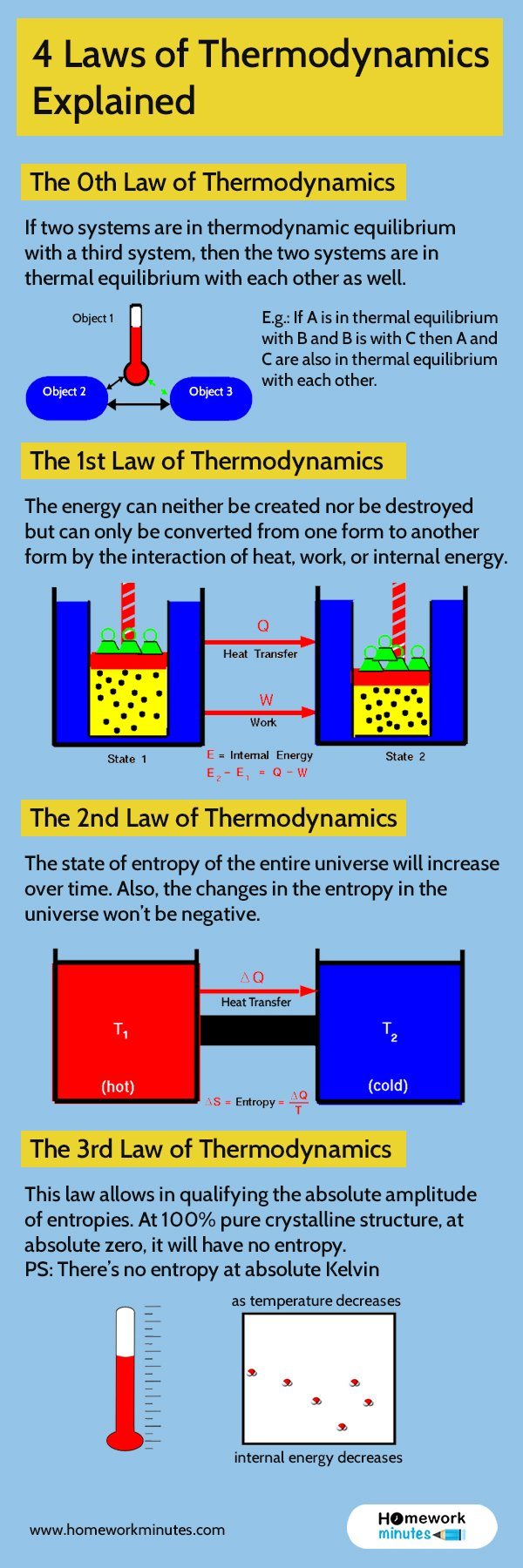# 4 Laws of Thermodynamics | Science GuidePrimarily three laws of thermodynamics explain physical quantities like temperature, entropy, and energy. It characterizes thermodynamic systems at thermodynamic equilibrium. These laws are essential as they define how these quantities change under particular circumstances. However, there is another law added known as “Zeroth Law” to establish thermal equilibrium. Let’s discuss all the laws one by one.

## The 0th Law of Thermodynamics

If two systems are in thermodynamic equilibrium with a third system, then the two systems are in thermal equilibrium with each other as well. The zeroth law helps in defining the concept of temperature. Since systems in thermal equilibrium with each other have the same temperatures.

E.g., If A is in thermal equilibrium with B and B is with C then A and C are also in thermal equilibrium with each other

## The 1st Law of Thermodynamics

Energy can neither be created nor be destroyed but can only be converted from one form to another form by the interaction of heat, work, or internal energy. Thus the total energy of an isolated system is constant.

If the system has a definite temperature, then the total energy will have three components. If the system is in motion, then there will be kinetic energy. The system has potential energy if it has an imposed force field. The establishment of the concept of internal energy differentiates the first law of thermodynamic from the general law of conservation.

## The 2nd Law of Thermodynamics

The second law of thermodynamics implies the existence of a quantity. This quantity is called the entropy of the thermodynamic system. The state of entropy of the entire world will increase over time. Also, the changes in the entropy in the universe won’t be negative. It indicates the irreversibility of the natural processes. This law applies to a wide variety of reversible or irreversible processes. Reversible processes are convenient theoretical fiction but do not occur in nature. A famous example is the transfer of heat by radiation or conduction.

## The 3rd Law of Thermodynamics

This law allows in qualifying the absolute amplitude of entropies. At 100% pure crystalline structure, at absolute zero, it will have no entropy. The system is in a state with minimum thermal energy at zero temperature. In general, it can be applied to several systems like glass. Since glass might have more than one minimum microscopically distinct energy state.

PS: There’s no entropy at absolute Kelvin

### Related Questions

Ihp 525 Quiz One

Precalculus/Calculus

Shakespeare Quiz Theatre Appreciation, Summer B 2019-20 ?

A 10 MV Signal Source Having an Internal Resistance of 100 K?

Ecet 330 Homework / Lab

The Assignment (2–3 Pages)

Aspen n510 2019 August All Assignments Latest (No Week 6 Assignment)

Implementing Basic Security Management Is an Important Task For